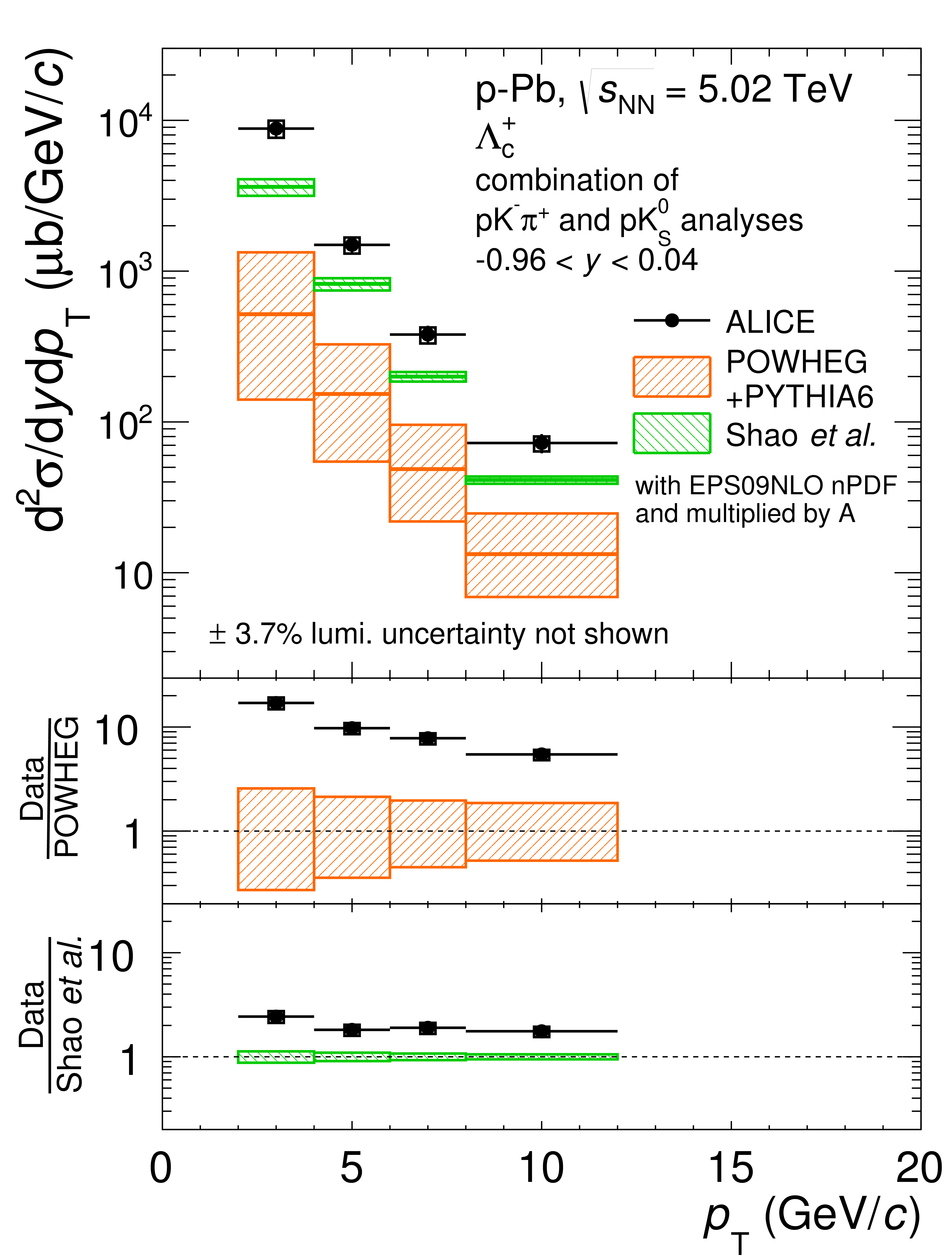# Figure 9

 Prompt \Lcplus~baryon $\pt$-differential cross section (average among different decay modes and analyses) in \pp collisions at \sqrts $= 7$ \tev in the transverse momentum interval $1 < \pt < 8$~\gevc (left) and in \pPb collisions at \sqrtsNN $= 5.02$ \tev in the transverse momentum interval $2 < \pt < 12$~\gevc (right). The statistical uncertainties are shown as error bars and the systematic uncertainties are shown as boxes. See text for details of the procedure to average the different decay channel measurements reported in Fig.~\ref{fig:CrossSection}. Comparisons with GM-VFNS calculations~, {\sc powheg} event generator~ and with Lansberg and Shao predictions~ for \pPb (see text for details) are also shown.